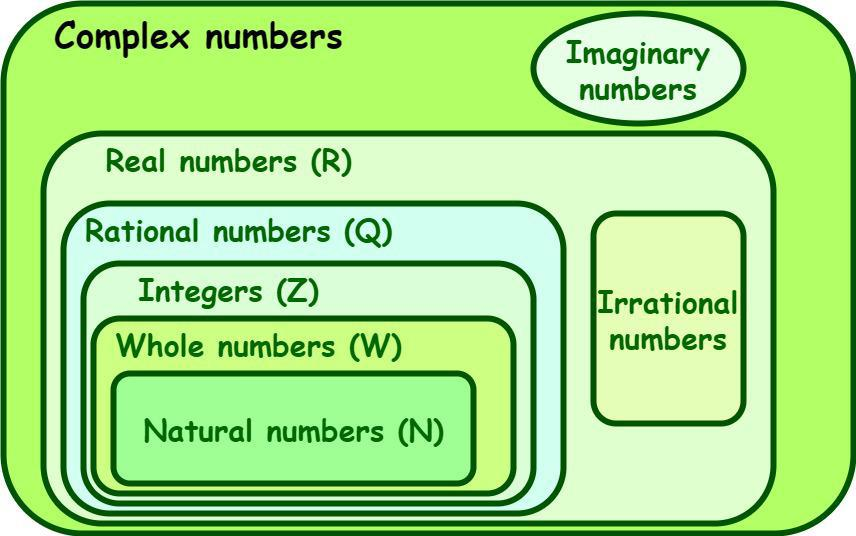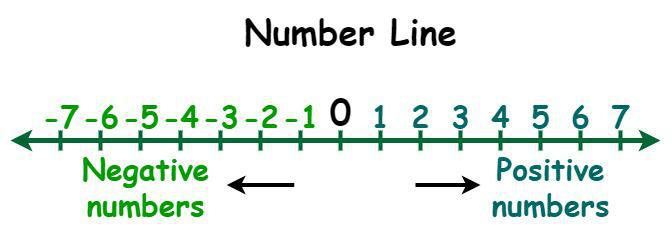GFG App
Open AppBrowser
Continue

# How to know if a function is positive/negative and how to know if it’s fractional?

In mathematics, a number system is a system of expressing numbers in various forms through writing. It is also often called the numeral system, which represents numbers by consistently using digits or other symbols. We have different types of number systems, such as a binary number system, an octal number system, a decimal number system, and a hexadecimal number system. Digits from 0 to 9 are used to represent the number systems. For example, a binary number system is represented by using the digits 0 and 1. A number is defined as a mathematical value that is used to count, measure, and also aid in performing various arithmetical calculations. There are different types of numbers, such as natural numbers, whole numbers, integers, rational and irrational numbers, etc.

## Numbers System

In math, a number is an arithmetic value that is used to count, measure, and also aid in performing various mathematical calculations. In a number system, a number is classified into various types based on its properties and representation on the number line. The different types of numbers are natural numbers, whole numbers, integers, rational and irrational numbers, and so on. The below diagram represents the sets of various types of numbers. The magnitude of a number expresses the size of the quantity, which can be either large or small.## How to know if a function is positive/negative and how to know if it’s fractional?

Positive number: A positive number is considered a number that is greater than “zero”. A plus symbol (+) can be placed in front of positive numbers, but we generally do not use it. So, we consider the number with no sign in front of it to be a positive number. Some examples of positive numbers are 2, 8, 15, 97, 112, 250, etc.

Negative number: A negative number is considered a number that is less than “zero”. Generally, a negative number is identified by the minus sign in front of it. Some examples of negative numbers are -221, -137, -85, -32, -14, -3, and so on.Now, let us consider a function y = f(x). Now, the function is said to be positive if the value of y is greater than zero; otherwise, it is a negative function, i.e., the value of y is less than zero. Graphically, a function is said to be positive if all the values of y are above the x-axis and it is said to be negative if all the values of y are below the x-axis.

### Rational/Fractional function:

A rational or fractional function is one with the form,

p(x)/q(x)

where p(x) and q(x) are polynomials and q(x) is not equal to zero.

Some of the examples of fractional functions are 1/(2x+3), (5x-7)/(11x2+19), (7x-3)/5, etc.

## Solved Examples on Numbers System

Example 1: Identify natural numbers among the following given numbers.

a) -34  b) 113  c) 0

Solution:

a) -34 is a negative number, so it is not a natural number.

b) 113 is a natural number.

c) 0 is not a natural number.

Example 2: Is √17 a rational number?

Solution:

No, √17 is not a rational number. The value of √17 is 4.123105625617661… It is a non-terminating and non-recurring decimal. So, √17 is not a rational number.

Example 3: What is the value of √-49?

Solution:

√(-49) = √(49)(-1)

= √(49) × √(-1)

We know that √(-1) = i

So, √(-49) = 7i.

Example 4: What is the decimal value of 3/5?

Solution:

To convert 3/5 into a decimal multiply the numerator and the denominator with 2, such that we obtain 10 in the denominator.

(3/5) × (2/2) = 6/10.

Now, convert this fraction with a denominator of 10 to a decimal by shifting the decimal point to one place towards the left.

6/10 = 0.6.

Hence, the decimal value of 3/5 is 0.6.

Example 5: Identify integers among the following given numbers.

a) √18/√2 b) -54.09 c) 12 d) 3/7

Solution:

a) √18/√2 = √(18/2)

= √9

= 3

3 is an integer, so, √18/√2 is an integer.

b) -54.09 is a decimal number. So, it is not an integer.

c) 12 is an integer

d) 3/7 is a fraction. So, it is not an integer.

## FAQs on Number System

Question 1: What is a Number System? What are the different types of Numbers in Number Systems?

In mathematics, a number system is a system of expressing numbers in various forms through writing. It is also often called the numeral system, which represents numbers by consistently using digits or other symbols. We have different types of number systems, such as a binary number system, an octal number system, a decimal number system, and a hexadecimal number system.

Question 2: What is meant by Real Numbers and give some examples?

A real number is a union of rational and irrational numbers and is expressed by the letter “R”. Irrational numbers, rational numbers, repeating decimals, terminating decimals, integers, whole numbers, natural numbers, and so on. are subsets of the set of real numbers. Some of the examples of real numbers are -6, √5, π, 8.9, 4/9, 0, 7, and so on.

Question 3: What are Natural Numbers and Whole Numbers?

Natural numbers are a set of positive integers from 1 to infinity that is expressed by the letter “N.” They are also known as “counting numbers”. The set of natural numbers is N = {1, 2, 3, 4, 5, ……….}. Some of the examples of natural numbers are 4, 23, 38, 76, 118, etc.

Whole numbers are the set of zero and all natural numbers and are expressed by the letter “W.” They are a set of non-negative integers and the set of whole numbers is W = {0, 1, 2, 3, 4, 5, ……….}. Some of the examples of whole numbers are 0, 5, 21, 61, 90, etc.

Question 4: What is meant by a Complex Number?

A complex number is a combination of a real number (R) and an imaginary number. It is represented as z = a + ib, where “a and b” are real numbers and “i” is an imaginary unit whose value is equal to √(-1). Some of the examples of complex numbers are 1-2i, 1 +√5i, 2+3i, 5+8i, etc.

Question 5: Define a positive number and a negative number.

Positive number: A positive number is considered a number that is greater than “zero”. A plus symbol (+) can be placed in front of positive numbers, but we generally do not use it. So, we consider the number with no sign in front of it to be a positive number. Some examples of positive numbers are 2, 8, 15, 97, 112, 250, etc.

Negative number: A negative number is considered a number that is less than “zero”. Generally, a negative number is identified by the minus sign in front of it. Some examples of negative numbers are -221, -137, -85, -32, -14, -3, and so on.

Question 6: What do we mean by a fraction in Mathematics?